## "The Fibonacci Spiral and the Shape of the Distal Femoral Articulating Surface of the Knee"

### Rene C Catan1*, Nino Ishmael Pastor2

1Southwestern University School of Medicine, Cebu City, Philippines

2Gullas College of Medicine, Cebu City, Philippines

*Corresponding author: Rene C Catan, Southwestern University School of Medicine, Cebu City, Philippines

Received Date: 03 May, 2020, 2020; Accepted Date: 10 May, 2020; Published Date: 16 May, 2020

#### Abstract

Fibonacci spiral is widely identified in non-biological fields such as mathematics, architecture and art, but not in human biology; an understanding of its functional role in human anatomy may be essential in making further advances in biomedical engineering specifically knee implant design. This study aims to explore whether the Golden Ratio and Fibonacci Spiral approximate the shape of the distal femoral articular surface of the knee. Twenty-three 3D CT scan images of the distal knee were digitalized in 2D sagittal views and best fitted over with Fibonacci spirals computed from the knee antero-posterior dimensions. The differences between the means of the radii of the Fibonacci Spiral and the digitalized images on 5/6 subsectors where not significant at p<0.05 using Krus-Wallis Test covering 84% of the distal articulating surface. With the Fibonacci presenting three independent axes described a “L” shaped on the sagittal plane, these findings affirm the fixed, flexion-extension axes of knee kinematics.

Introduction

The golden ratio, also known as the golden section or golden proportion, is obtained when two segment lengths have the same proportion as the proportion of their sum to the larger of the two lengths. The value of the golden ratio, which is the limit of the ratio of consecutive Fibonacci numbers, has a value of approximately 1.618 which is most commonly represented by the Greek Letter Phi (φ). The Fibonacci sequence is the sum of the two numbers that precede it. So, the sequence goes: 0, 1, 1, 2, 3, 5, 8, 13, 21, 34, and so on. The mathematical equation describing it is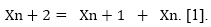Furthering this observation, shapes can be created based upon length measurements of the Fibonacci numbers in sequence. Rectangles created using consecutive numbers from the Fibonacci sequence can be divided into equally sided squares of such numbers as well. The length of a single side can be divided into smaller values of the Fibonacci sequence. Connecting the diagonals of each of the squares creates a spiral, known as the Golden Spiral (Figure 1). This pattern is seen in several different forms in nature .

Classically, the Golden Spiral approximates the spiral of the human ear (Figure 2), the shell of a nautilus (Figure 3).

Human Anatomy

In 1973, Dr. William Littler proposed that by making a clenched fist, Fibonacci’s spiral can be approximated (Figure 4). However, due a lack of statistical and well documented empirical data, accurate representations of the golden spiral could not be readily ascertained. Hamilton and Dunsmuir (2010) confirmed that the phalangeal length ratio data obtained from their subjects compared to those that were almost arbitrarily listed by Littler in 1973 , were in fact comparable and approximated a Lucas series, which essentially underscores a Fibonacci sequence in that the sum of the first two lengths equaled the third length the Fibonacci value of (φ) of 1.618 .

Fibonacci Series and the underlying Lucas series are observed in several aspects of life on planet earth and within the cosmos. Although widely identified in non-biological fields such as architecture and art, it has not been well explored in the human biology. More research needs to be performed to explore its physiological role in biology. Understanding its functional role may be a keystone to making quantum advances in several fields such as artificial intelligence, biomedical engineering designs, and human regeneration, amongst others.

This study aims to explore whether the Fibonacci Sequence and Fibonacci Spiral approximate the shape of the distal femoral articular surface of the knee.

Methodology

Twenty-three 3D CT Scan images were downloaded from the internet and copied into the PowerPoint Microsoft Office program of a computer. The following criteria were used in choosing the images:

1.             Knee images must have at least 2 views: antero-posterior and lateral.

2.             Images must show the distal anterior and posterior femoral cortices.

3.             Distal articulating surface including the distal femoral condyle must be traceable on the on the lateral view

Each image was assigned a random number from a Google random number generator (range: 50 to 65) representing the AP dimensions (cm) of the knee. The images were then digitalized in bundled 3D Paint software application. The distal femur was cropped out. The outline of the 3D lateral image of the distal knee was traced using stylus (SPEN-HP-01) and the rendered image was then copied into a separate PowerPoint file. Images were locked at 1:1 aspect ratio. Each digitalized image was copied into one ppt slide each (slide 1-23).

A line (X) was draw parallel to the anterior cortex of the digitalized outlined image of the knee. And another line (Y) was drawn at the posterior tip of the distal femoral condyle and parallel to the line X. The three images were arranged in group image (W) formatted with aspect ratio 1:1 (Figure 5). Each downloaded, digitalized and grouped image was copied into one ppt slide each (slide 1-23).

Drawing the Fibonacci Spiral (Figure 6)

Note: The pattern for creating the Fibonacci Spiral follows the Fibonacci sequence of numbers are generated by setting F0 = 0, F1 = 1, and then using the recursive formula Fn = Fn-1 + Fn-2 to get the rest. Thus, the sequence begins: 0, 1, 1, 2, 3, 5, 8, 13, 21, … This sequence of Fibonacci numbers arises all over mathematics and also in nature.

On a separate file a square (b1) with sides (1x1) was inserted with no fill with dashed outline. Another square (b2) was drawn alongside b1 thus creating a 1x2 rectangle (r1). A 2x2 box (b3) is inserted alongside the 1x2 rectangle (r1) following the sequence of 1,1,2 forming a 2x3 rectangle (r2). The sum of the previous two numbers in the sequence being 1 and 2 has a sum of 3, therefore, a fourth square (b4) with a 3x3 configuration is drawn and is attached alongside rectangle 2 (r2) creating third rectangle (r3) with a 3x5 configuration. Then a fifth 5x5 square (b5) is inserted alongside the preceding rectangle (r3) forming a 5x8 rectangle (r4). And the final square (b6) is an 8x8 square alongside the (r4) creating a an 8x13 rectangle (r5).

The Fibonacci Spiral is then drawn to approximate the golden spiral using quarter-circle arcs on each square derived from the Fibonacci sequence.

Fibonacci Spiral Template

The distance between the parallel lines x and y is the Antero-posterior dimensions (AP) in cm of the distal femoral articulating is referred to as A1 corresponding to the measurement of square b6 in Figures 6, 7.

The Fibonacci sequence describes the relationship with the Golden Rectangle as follows: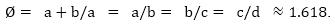where a=b6; b=b5, c=b4, d=b3, e=b2, f=b1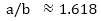b6/b5≈1.618.

Therefore,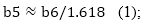where b6=A1, b5= A2.

Subsequently,

b/c≈ 1.618

b5/b4≈1.618

Therefore,      b4≈b5/1.618      (2), b4= A3.

The rest of the squares can be calculated by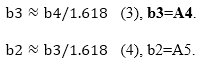A1’, A2’, A3’, A4” are the Axes of Rotation (AoR) of the quarter circle in each square. Black arrows represent distance covered by the arc from the AoR in each square.

The center of rotation for each arc drawn in each square and hereto referred as A1’, A2’, A3’, A4’and A5’. The arcs are formed one quarter of circle shaped by the radius that equal to the dimension of the side of the square. These are referred hence ArcA1, ArcA2, ArcA3, ArcA4 and ArcA5 respectively (Figure 8).

Each arc is further subdivided into two subgroups thus we have the following: A2.1, A2.1, A3.1, A3.2, A4.1, and A4.2, respectively. There are six (6) subsectors (Figure 9).

Posterior tilt of the template if necessary is measured to allow best fit of outlines of the template over the digitalized image. Increments of 5 degrees up to 20 degrees is merged into the template with its vertex at A2’ (Figure 9).

All lines in figure 9 are formatted, grouped and its aspect ratio locked at 1:1. This template (“T”) hence is used to ascertain the shape of the distal femoral articulating surface.

Procedure

The slide is set at 116% magnification to mark actual centimeter values on the computer screen. The digitalized image of the distal femur is enhanced or reduced with aspect ratio locked at 1:1 based on its actual AP dimension in centimeters.

Template “T” is copied into each slide of the rendered image (slide 1-23) and its A1 dimension enhanced or reduced to equalize or closely approximate the AP dimension of the digitalized image.

Template T is set on the digitalized image of the distal femur to approximate fit of ArcA2, ArcA3 and ArcA4 over the articulating surface of the femur. Posterior or anterior rotation of the template is permitted to allow as much as possible 100% fit of the two images. Once it is acceptable the two images are grouped.

The values of A2, A3, A4 and A5 of template “T” are determined using equations (1), (2), (3), and (4) which are equal to the dimensions of the squares and the radius of the arc, respectively.

On of each subsector: A2.1, A2.2, A3.1, A3.2, A4.1 and A4.2 the digitalized image of the femur is measured from the center of the arc of rotation on template “T” to the maximum distance protracted by the image (radius) (Figure 10).

The preceding procedure is performed on each slide (Slide1 to 23) and the values are documented on excel file.

Statistical Analysis

The mean of the radius of template “T” (A2, A3, A4) are compared to the mean of the radius on each subsector using Mann-Whitney/Wilcoxon Two-Sample Test (Kruskal-Wallis test for two groups), values p < 0.05 is significant.

1)            A2 cf A2.1

2)            A2 cf A2.2

3)            A3 cf A3.1

4)            A3 cf A3.2

5)            A4 cf A4.1

6)            A4 cf A4.2

Results

Data Comparing the Shapes (Tables 1 to 4)

Discussion

Geometry of the Femoral Condyles

The tibiofemoral joint is formed by the distal end of the femur and the proximal surfaces of the tibia. The distal aspect of the femur has two surfaces. Both are convex, asymmetrical, saddle-shaped condylar surfaces are coated with cartilage. They are separated by a U-shaped notch. The femur viewed laterally, is flattened in its anterior surface and curved on its posterior aspect. The medial femoral condyle has a smaller transverse diameter but longer longitudinal length than the lateral condyles.

As early as the late 19th century, the axis of knee ﬂexion and extension was derived from the geometry of the femoral condyles, by analysis of true sagittal plane sections through the femoral condyles.

The femoral condyles were described as spirals and the changing curvature of the condyles seen on sagittal sections results in an axis that moves as the knee ﬂexes and extends. This was described as “the instant center of motion” moving along a predictable curved pathway during knee ﬂexion.

Fick in 1911 reanalyzed the condyle shapes by using 3 dimensions and concluded that the ﬂexion-extension axis of the knee was offset that resulted in a single, ﬁxed axis, rather than an instant center.

Contemporary movement toward the concept of a ﬁxed, ﬂexion-extension axis began in the ﬁeld of total knee arthroplasty. Use of the epicondylar axis to align the prosthesis during surgery indicated that a conceptual shift was taking place in the view of knee kinematics at this time.

In vitro research has led to the development of a model of the tibiofemoral joint with 3 independent axes of motion. One, the posterior condylar axis is effective from approximately 15° to 150° of knee ﬂexion. It closely approximates the epicondylar line and is offset from the sagittal plane by 7° . Two, as the knee reaches extension, the axis of motion shifts from the posterior condylar axis to the larger distal condylar axis coupled with an anterior shear motion of the the tibia on the distal femur . The reverse occurs from full extension to the first 20° of flexion where there is posterior gliding of the tibia until it reaches posterior condylar axis. And three is the longitudinal axis of rotation of the knee in coronal plane .

Fibonacci Spiral

The investigator recreated a Fibonacci Spiral based on the antero-posterior dimesion of the distal femur on the sagittal plane (A1). Subsequent measurements of of the Golden Rectagle were derived by dividing by the value with Ø = 1.618 to generate template “T”. The latter represented the “idealized” shape of the distal femoral articulating surface.

Thru best-fit technique and by statistical analysis (Krus-Wallis H, p<0.05) comparing template “T” with the digitalized femoral image, the differences of the means of all subsectors (A2.1, A2.2, A3.1, A4.1 A4.2) are p>0.05. Therefore, the null hypothesis is accepted in all subsectors except A3.2 where p value= 0.0375. This investigator has shown that the distal femoral shape is consistent with a Fibonacci Spiral (5/6 subsectors or 84%). Furthermore, the Fibonacci Rectangle is rotated posteriorly from the horizontal axis of the distal anterior femoral shaft by an average of 21.3°.

Knee Kinematics and Fibonacci Spiral Model

Based on Fibonacci Spiral Model, A2’ and A3’ represent the larger condylar and smaller posterior condylar axes, respectively. With posteior tilt of Template “T” to accommodate best fit of Fibonacci Spiral over the digitalized image, the axes A2 and A3 are not aligned horizontally but are off set by about 21°. This offset translates to movement of the tibial perpendicular to the axes.

Beginning at full extension (0°) of the tibio- femoral joint in alignment with A2’ the tibia glides posteriorly and distally to about 21° flexion until the tibial axis reaches the posterior condylar axis (A3). The axis of rotation shifts distally and posteriorly from A2’ to A3’ to reach 110° (20°+90°). In order to achieve flexion beyond 110° the axis of rotation following the Fibonacci Spiral Model then shifts to more posterior and more superior condylar axis at A4’ but ith a shorter arc. The axes hence follow an “L” shaped.

Although it is beyond the scope of this investigation, it is worth mentioning that the medial and collateral ligaments are taut at complete extension, permitting no varus or valgus motion and no rotation on the axial plane. The anterior fibers of the medial collateral ligaments incline forward as they descenf on the tibia, blocking its rotation. After 20° of flexion the ligaments become relaxed permitting both gliding and axial rotation. The degree of rotation increases as flexion increases and is maximum at full extension .

Conclusion

The Fibonacci Spiral approximate the shape of the distal femoral curvature; and shifting axes explains the knee flexion extension movement through the sagittal plane.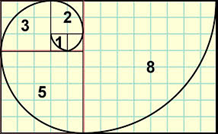Figure 1: Golden Spiral fashioned out from Fibonacci sequence of numbers.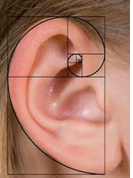Figure 2: Fibonacci Spiral approximates the spiral of a human ear.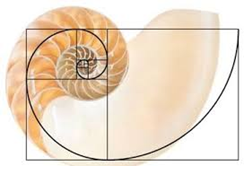Figure 3: Shell of a Nautilus approximates a Golden Spiral.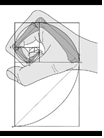Figure 4: Fibonacci Spiral approximated by a clenched fist.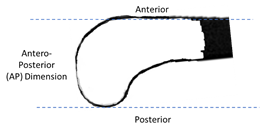Figure 5: Antero-posterior ((AP) Dimensions of digitalized image distal femur.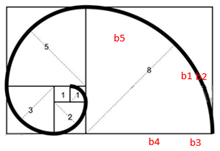Figure 6: Fibonacci Spiral.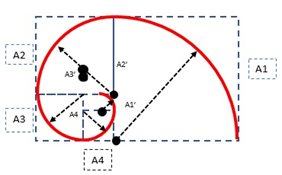Figure 7: Drawing the Fibonacci Spiral. A1 corresponds to b6; A2=b5; A3=b4; A4=b3 and A5=b2.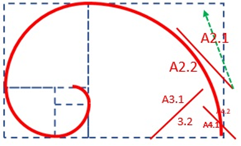Figure 8: Six subsectors: A2.1, A2.2, A3.1, A3.2, A4.1 and A4.2.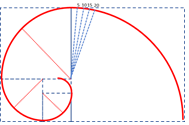Figure 9: Template “T” with angulation showing degrees of posterior tilt with vertex at A2’.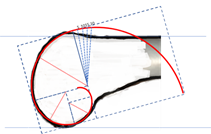Figure 10: Approximation of Fit of Template “T” on Digitalized Image.

Note: Arrow indicates distance from center of rotation to the maximum distance protracted by the digitalized image on subsector.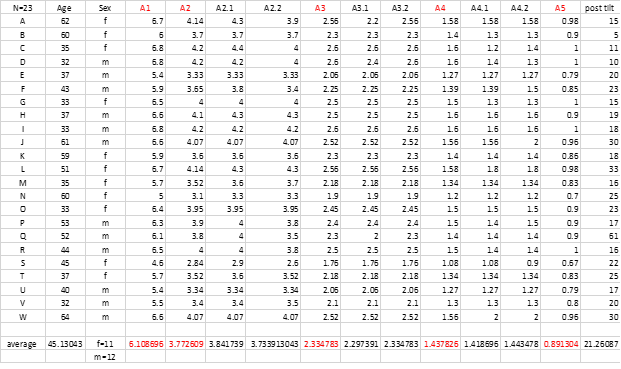Table 1: Data Comparing the Shape of the Distal Femoral Articulating Surface with Fibonacci Spiral (N=23).

 Descriptive Statistics for Each Value of Crosstab Variable Observed Total Mean Variance Std Dev Female 11 510 46.3636 150.2545 12.2578 Male 12 528 44 123.4545 11.111 Minimum 25% Median 75% Maximum Mode Female 33 35 45 60 62 33 Male 32 35 41.5 52.5 64 32

Table 2: MEANS Age by Sex.

 Obs Total Mean Variance Std Dev 23 1038 45.1304 131.482 11.4666 Minimum 25% Median 75% Maximum Mode 32 35 43 59 64 33

Table 3: MEANS Age (Male & females).

 Comparison of Means Krus-Wallis H (Equivalent to Chi Square) Degrees of Freeedom p Value p < 0.05 A2 cf A2.1 21.7272 13 0.0597 not significant A2 cf A2.2 20.5623 15 0.1515 not significant A3 cf A3.1 20.9044 14 0.1014 not significant A3 cf A3.2 22.0000 12 0.0375 significant A4 cf A4.1 15.0578 12 0.2383 not significant A4 cf A4.2 16.4247 10 0.0881 not siginififant Mann-Whitney/Wilcoxon Two-Sample Test (Kruskal-Wallis test for two groups)

Table 4: Comparison of Means Template “T” cf Distal Femoral Shape.

Citation: Catan RC, Pastor NI (2020) The Fibonacci Spiral and the Shape of the Distal Femoral Articulating Surface of the Knee. Human Anat Physiol Open Acc 3: 102. DOI: 10.29011/HPAOA.100002